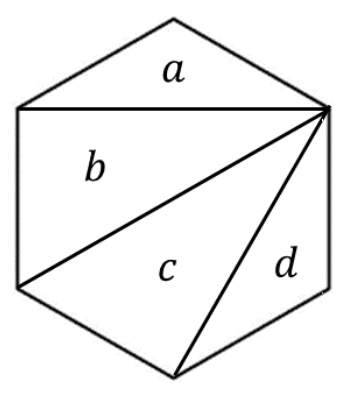시간 제한메모리 제한제출정답맞힌 사람정답 비율
1 초 512 MB41124419258.359%

## 문제

A regular n-sided polygon P can be partitioned into n − 2 triangles by adding non-crossing line segments connecting two vertices of P. For example, a square can be partitioned into two triangles, a regular pentagon can be partitioned into three triangles, and a regular hexagon can be partitioned into four triangles. The resulting set of triangles is called a triangulation of P. There exist two or more triangulations of P if n is greater than three.

Once a triangulation T of P is chosen, the distance between two triangles a and b in T is defined to be the number of hops crossing the borders of two adjacent triangles when you travel from a to b. Note that you must stay inside the polygon P, at any time during this travel, that is, it is not allowed to hop crossing the border of P.

For example, the distance of a and d in the triangulation shown in Figure J.1 is three since the triangles, a, b, c, and d, should be visited to travel from a to d, and you have to hop three times crossing borders between triangles.Figure J.1 A triangulation of a regular hexagon

The diameter of a triangulation T is the maximum of the distances between all pairs of triangles in T. Write a program to find a triangulation of a regular n-sided polygon P whose diameter is the minimum over all possible triangulations and print its diameter.

## 입력

Your program is to read from standard input. The input starts with a line containing n (3 ≤ n ≤ 1,000,000), where n is the number of sides of the regular n-sided polygon.

## 출력

Your program is to write to standard output. Print exactly one line. The line should contain the minimum diameter of the triangulations of a regular n-sided polygon.

## 예제 입력 1

3


## 예제 출력 1

0


## 예제 입력 2

4


## 예제 출력 2

1


## 예제 입력 3

6


## 예제 출력 3

2